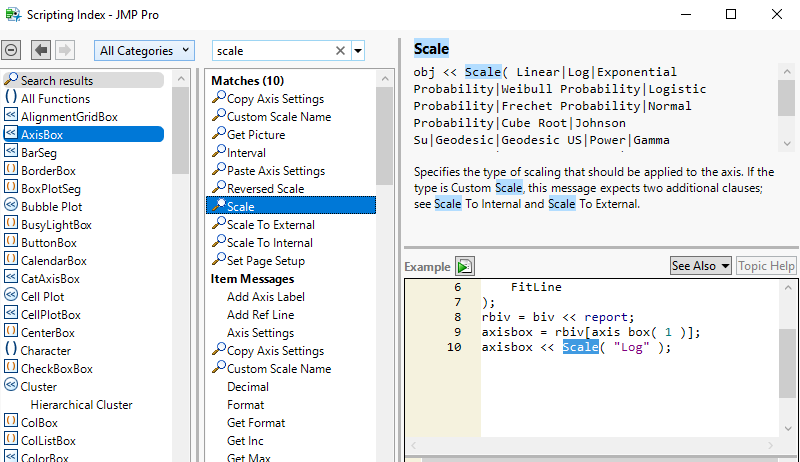Choose Language Hide Translation Bar
Highlighted

## editing variabilty chars in jsl

How can I change the scale of a variability plot, after it has been created. I need to create the plot first, then change the scale to log later.

Following is my current attempt, but it doesn't change to log plot.

``````cc2 = Variability Chart(
Y( :Data ),
X( :Trend, :Group ),
Std Dev Chart( 0 ),
Points Jittered( 0 ),
Show Box Plots( 1 ),
);

if (log_plot_needed,
//change scale of plot to log
report(cc2)[scalebox(1)] << set scale({scale("Log")}; //this line is not correct.
);``````

Also, This may go outside the scope of the question, but is there a way to determine how to change items for any graph?  I frequently use the "save script" option on graphs to help me find the jsl code, but this only helps when I create the graph all at once.  I'm not sure how to change the graph later without looking into each one.

1 ACCEPTED SOLUTION

Accepted Solutions
Highlighted

## Re: editing variabilty chars in jsl

Here is the corrected script, and a pointer to the reference in the Scripting Index.  The change is made to the AxisBox() not the ScaleBox()

``````names default to here(1);
dt=open("\$SAMPLE_DATA/big class.jmp");

cc2 = Variability Chart(
Y( :height ),
X( :sex ),
Std Dev Chart( 0 ),
Points Jittered( 0 ),
Show Box Plots( 1 ),
);
log_plot_needed=1;
if (log_plot_needed,
//change scale of plot to log
report(cc2)[axisbox(1)] << scale("Log");
);``````Jim
2 REPLIES 2
Highlighted

## Re: editing variabilty chars in jsl

Here is the corrected script, and a pointer to the reference in the Scripting Index.  The change is made to the AxisBox() not the ScaleBox()

``````names default to here(1);
dt=open("\$SAMPLE_DATA/big class.jmp");

cc2 = Variability Chart(
Y( :height ),
X( :sex ),
Std Dev Chart( 0 ),
Points Jittered( 0 ),
Show Box Plots( 1 ),
);
log_plot_needed=1;
if (log_plot_needed,
//change scale of plot to log
report(cc2)[axisbox(1)] << scale("Log");
);``````Jim
Highlighted

## Re: editing variabilty chars in jsl

Thank you.  That is exactly what I needed.  I didn't know that the Scripting Index was there, but it should be very usefull in the future.

Article Labels

There are no labels assigned to this post.